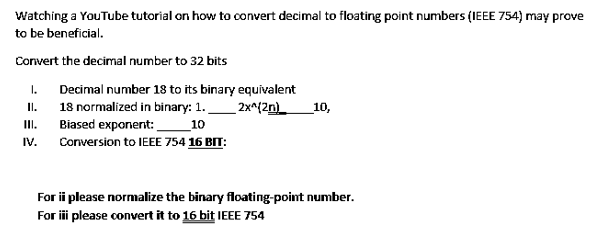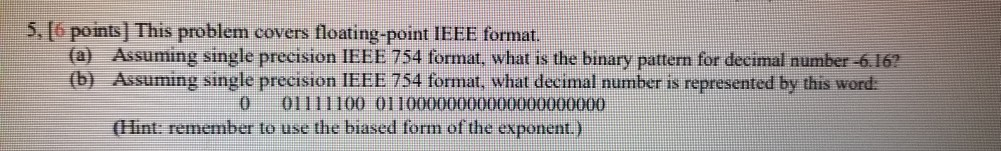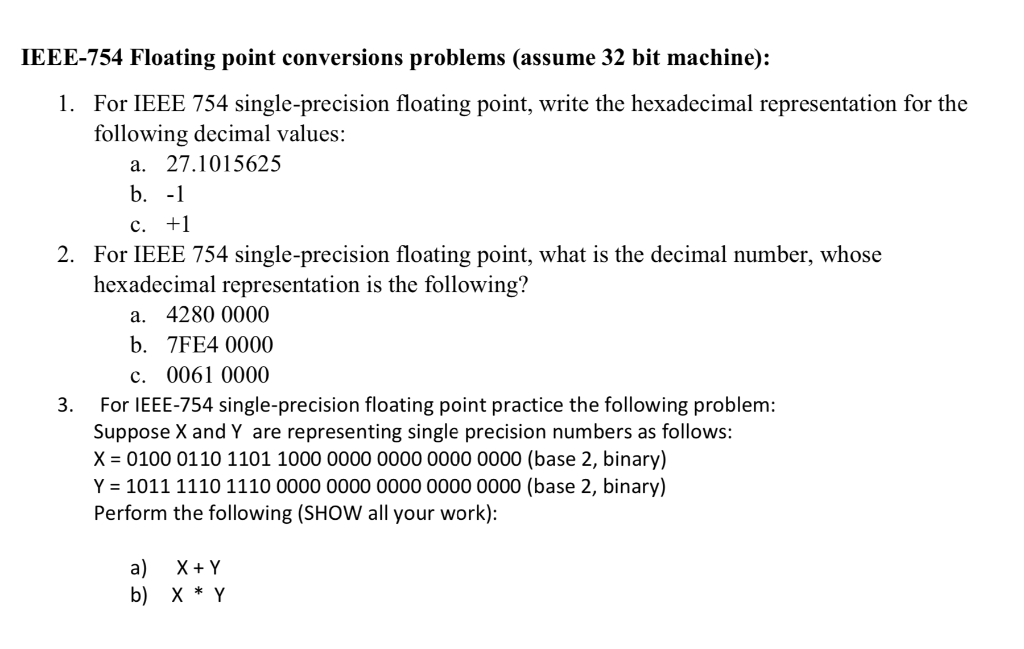Question

# convert -1.525*10^-5 to binary using IEEE 754 std

convert -1.525*10^-5 to binary using IEEE 754 std

We need at least 10 more requests to produce the answer.

0 / 10 have requested this problem solution

The more requests, the faster the answer.

All students who have requested the answer will be notified once they are available.

#### Earn Coins

Coins can be redeemed for fabulous gifts.

Similar Homework Help Questions
• ### Watching a YouTube tutorial on how to convert decimal to floating point numbers (IEEE 754) and...Watching a YouTube tutorial on how to convert decimal to floating point numbers (IEEE 754) and normalisation may prove to be beneficial. Watching a YouTube tutorial on how to convert decimal to floating point numbers (IEEE 754) may prove to be beneficial Convert the decimal number to 32 bits I Decimal number 18 to its binary equivalent I. 18 normalized in binary: 1.-2刈2n) II Biased exponent: 10 IV. Conversion to EE 754 16 I: 10, For ii please normalize the...

• ### The value shown below is represented using the IEEE 754 single precision format. Convert to a...

The value shown below is represented using the IEEE 754 single precision format. Convert to a signed decimal number. 11101010111010000000000000000000

• ### Consider the following 32 bit binary representation of the value using IEEE 754 single precision floating...

Consider the following 32 bit binary representation of the value using IEEE 754 single precision floating point representation. Show the corresponding signed number in decimal. 01000001001010100000000000000000

• ### convert the following decimal values to IEEE 754 single precision. when converting the fractional part to...

convert the following decimal values to IEEE 754 single precision. when converting the fractional part to binary, stop when the total number of bits in the mantissa is 12. Give your answer in hex. 1. 48.0 2. 11.11

• ### Convert 0.0075 to equivalent IEEE 754 FP representation

Convert 0.0075 to equivalent IEEE 754 FP representation.Can someone show me the steps on how to do this?!? Thank you!

• ### Show the IEEE 754 binary representation of the numbers 0.75 and 0.50. Multiply the two numbers...

Show the IEEE 754 binary representation of the numbers 0.75 and 0.50. Multiply the two numbers in binary Check for overflow/underflow

• ### 5, [points] This problem covers floating-point IEEE format. (a) Assuming single precision IEEE 754 format, what...5, [points] This problem covers floating-point IEEE format. (a) Assuming single precision IEEE 754 format, what is the binary pattern for decimal number -6.16? (b) Assuming single precision IEEE 754 format, what decimal number is represented by this word: 0 01111100 01100000000000000000000 (Hint: remember to use the biased form of the exponent.)

• ### 1 please IEEE-754 Floating point conversions problems (assume 32 bit machine): 1. For IEEE 754 single-precision...1 please IEEE-754 Floating point conversions problems (assume 32 bit machine): 1. For IEEE 754 single-precision floating point, write the hexadecimal representation for the following decimal values: a. 27.1015625 b.-1 2. For IEEE 754 single-precision floating point, what is the decimal number, whose hexadecimal representation is the following? a. 4280 0000 b. 7FE4 0000 c. 0061 0000 3. For IEEE-754 single-precision floating point practice the following problem: Suppose X and Y are representing single precision numbers as follows: X 0100...

• ### For IEEE 754 single precision floating point, what is the number, as written in binary scientific...

For IEEE 754 single precision floating point, what is the number, as written in binary scientific notation, whose hexadecimal representation is the following? Show your work B350 0000 (hex)

• ### This problem covers floating-point IEEE format. (a) Assuming single precision IEEE 754 format, what is the...

This problem covers floating-point IEEE format. (a) Assuming single precision IEEE 754 format, what is the binary pattern for decimal number -6.16? (b) Assuming single precision IEEE 754 format, what decimal number is represented by this word: 0 01111100 01100000000000000000000 (Hint: remember to use the biased form of the exponent.)